# The KCRC TechNet Puzzles For 2018

## The KCRC TechNet Puzzle for December 26, 2018

In a circuit containing only ‘pure’ inductance…

A.  the voltage and the current are in phase
B.  the voltage and the current are separated by 180°
C.  the voltage lags the current by 90°
D.  the voltage leads the current by 90°.

And now for the answer to our December 26, 2018 TechNet Puzzle

(It is in “Invisotext” and will be visible if you highlight the area below!)

Well, you pretty much have to know how inductance and capacitance work to answer this one, or you can just memorize which leads and which lags. When you apply a voltage potential across the plates of a capacitor a current rapidly flows to charge each opposing plate with the opposite charge, so current “leads” the voltage OR voltage “lags” the current. When a voltage potential is placed on an inductor, an opposing current is induced in the inductor, that opposes a current from flowing – so in an inductor voltage “leads” current OR current “lags” voltage.

The phase difference between an alternating power source’s voltage and its current is determined by the resistance and the reactance (either inductive reactance or capacitive reactance) for that circuit. A circuit with a pure resistance has NO phase difference or 0 degrees. A complex impedance, possessing both resistance and reactance possess a phase difference between, but NOT equal to -90 to +90 degrees. A purely inductive reactance produces a phase difference of 90 degrees. Therefore, answer (D) “the voltage leads the current by 90 degrees is the correct answer!

(Here’s a nice article on the subject!)

.

## The KCRC TechNet Puzzle for December 12, 2018

What mode of signals is defined this way:
‘signals that are in phase on 2 or more conductors in a cable’

A. Common mode signals.
B. Data mode signals.
C. SSB mode signals.
D. Wide-band mode signals.

And now for the answer to our December 12, 2018, TechNet Puzzle

(It is in “invisotext” and will be visible if you highlight the area below!)

There are two kinds of “signals” possible on any given transmission line – the kind that most would prefer is called “Differential Mode”. This is when two conductor’s currents are 180 degrees out of phase with each other and local induced RF is canceled out by negative interference. The other mode is called “common mode”, often seen when your antenna is not resonant and provides n impedance different than your transmission line – this leads to a reflected standing wave, with a Voltage Standing Wave Ratio other than 1:1. This “common mode” signal has the same phase relationship as your other current and doesn’t interfere and your transmission line begins to act more like a bad antenna rather than a good transmission line.

Or, in short, the correct answer is “A” Common mode signals.

.

## The KCRC TechNet Puzzle for November 28, 2018

How long is the perimeter of a quad antenna?

A. ¼ wavelength
B. ½ wavelength
C. ¾ wavelength
D. 1 wavelength

And now for the answer to our November 28, 2018, TechNet Puzzle

(It is in “Invisotext” and will be visible if you highlight the area below!)

Unfortunately, you just have to know what a quad antenna is. It is a square-like piece of wire with its feed point at either the right-angle corner of the square or in the middle of one of the square’s leg.

Quad antennas are designed for full wavelength long wire wrapped into a square.

So, the correct answer is “D”, 1 wavelength.

## The KCRC TechNet Puzzle for November 14, 2018

At what frequency ‘will the third harmonic of a transmission on 14.1 MHz be found?

A. 28.2 MHz
B. 42.3 MHz
C. 47.4 MHz
D. 56.9 MHz
.

And now for the answer to our November 14, 2018 TechNet Puzzle

(It is in “Invisotext” and will be visible if you highlight the area below!)

Harmonics are integer (1, 2, 3,..) multiples of the original frequency, so the “first harmonic of a 14.1 MHz signal would be 14.1 MHz x 1, or the same 14.1 MHz frequency. The third harmonic of 14.1 MHz is 14.1 MHz x 3 or 42.3 MHz!

So, the correct answer is “B” 42.3 MHz!

## The KCRC TechNet Puzzle for October 24, 2018

What would you expect your ohm-meter to read connected to points “P” and “Q”?A. 0.9 ohms
B. 1.8 ohms
C. 2.0 ohms
D. 3.5 ohms

And now for the answer to our October 24, 2018 TechNet Puzzle

(It is in “Invisotext” and will be visible if you highlight the area below!)

Well, you have to remember that resistances in series add their individual resistances, while resistance in parallel is the inverse of their inverted sums. In other words, the 1 ohm and 5-ohm resistors wired in series are equivalent to a single 6-ohm resistor. The total resistance of a 3 ohm and a 6-ohm resistor wired in parallel is the inverse sum of 1/6 + 1/3 – the sum of 1/3 + 1/6 is 3/6. Its inverse is 6/3 or…

2

## The KCRC TechNet Puzzle for October 10, 2018

The most likely detrimental effect of a strong RF signal on a semi-conductor junction within audio equipment is…

A. reverse biasing
B. open-circuiting
C. rectification

And now for the answer to our October 10, 2018 TechNet Puzzle

(It is in “Invisotext” and will be visible if you highlight the area below!)

The biggest nuisance/noise stray RF in this situation would cause would be by the RF being rectified in a nonlinear way through a p-n or n-p junction of a semiconductor and injecting resulting audio frequency noise into an audio amplifying circuit.

## The KCRC TechNet Puzzle for September 26, 2018

In a radio wave that is defined as “horizontally polarized”, the magnetic component exists…

A. only in the vertical plane.
B. only in the horizontal plane
C. in both the vertical and horizontal planes
D. in neither the horizontal plane, nor the vertical plane, but at right angles to both planes in the direction of the pointing vector

And now for the answer to our September 26, 2018 TechNet Puzzle

(It is in “Invisotext” and will be visible if you highlight the area below!)

By definition, an electromagnetic wave’s polarization is the plane of the electric field, which is at a right angle to its simultaneous magnetic field. If the electromagnetic wave is said to be horizontal, its electric field is oriented horizontally and then its magnetic field must be oriented vertically, and vice versa – the direction of propagation, its pointing vector is located at right angles to both its magnetic field and its electric field.

With all that under our belts, it would seem as though a “horizontally polarized radio wave would have its magnetic field “only in the vertical plane”, as shown in answer “A”, which is the correct one.

## The KCRC TechNet Puzzle for September 12, 2018

A 3-element Yagi antenna is shown in this diagram. Which is the director?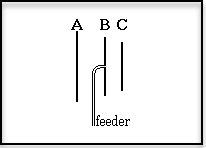A.  Element A
B.  Element B
C.  Element C
D.  None of the drawn elements is the director

And now for the answer to our September 12, 2018 TechNet Puzzle

(It is in “Invisotext” and will be visible if you highlight the area below!)

The way that the Yagi (or more accurately Yagi-Uda Array) works is by assembling a normal half-wave dipole connected to the antenna feed as the “driven element” (B), and then arranging two or more parasitic elements that are not electrically connected to anything else, but “float” free on a common mast. A parasitic element that is slightly longer than the half-wave dipole operates as a “reflector” (A), reflecting the dipole’s signal away from it, and one or more “director” (C) paralytic elements shorter than the driven half-wave dipole focusing the radio waves propagating from the antenna forward into a more directional signal.

So, obviously from that description choice “C” – Element C is the correct answer.

## The KCRC TechNet Puzzle for August 8, 2018

From where in a receiver’s stages is the automatic gain control usually obtained?

A. Detector.
B. First IF
C. Pre-amplifier
D. Attenuator

And now for the answer to our July 25, 2018 TechNet Puzzle

(It is in “Invisotext” and will be visible if you highlight the area below!)

Automatic Gain Control effectively gains or lowers your signal’s volume, to obtain similar volume levels for weak as well as strong signals. The usual method to accomplish this is a feedback mechanism to raise or lower an RF amplification stage based upon the final signal strength of your receiver. The best place to obtain final signal strength is right after the detector circuit. This voltage level is then used to determine the amplification factor of an RF amplifier stage or an IF stage.

So, the correct answer is “A” – a radio’s automatic gain control signal level is obtained around the detector stage.

## The KCRC TechNet Puzzle for July 25, 2018

The figure as shown here depicts:

##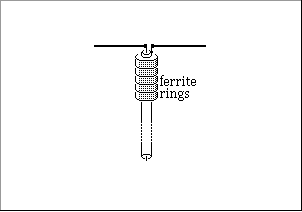A.   a down converter
B.   a static discharge device
C.   a choke balun
D.   an open wire transmission line

And now for the answer to our July 11, 2018, TechNet Puzzle

(It is in “invisotext” and will be visible if you highlight the area below!)

This is a W2DU-type 1:1 Balun/RF Choke Balun, plain and simple…
So, answer “C” is the right one!

## The KCRC TechNet Puzzle for July 11, 2018

Referring to the circuit below, the voltage across the resistor R is illustrated in which graph below: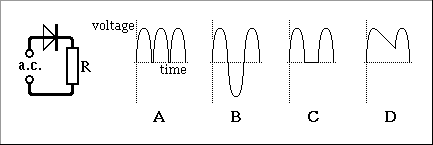And now for the answer to our June 27, 2018, TechNet Puzzle

(It is in “invisotext” and will be visible if you highlight the area below!)

A single diode cuts off current flowing in one direction, so half of the original sine wave should be conducted. That is, obviously, what is seen in graph “C”. Graph “A” would be seen by a bridge rectifier setup, and graph “B” would be seen if a rectifier was not present in the circuit.

## The KCRC TechNet Puzzle for June 27, 2018

What’s the difference between AFSK and FSK?

B.   AFSK is the Amateur equivalent of FSK
C.   AFSK is Audio FSK
D.   There is no difference, just different names for the same thing

And now for the answer to our June 27, 2018 TechNet Puzzle

(It is in “Invisotext” and will be visible if you highlight the area below!)

Well, the answer is relatively simple if you know what the acronym stands for. FSK is Frequency Shift Keying. It can have any number of distinct frequency shifts, the simplest implementation is two different frequency shifts, which let you implement your text in a binary form. For Radio Teletype (RTTY) the two frequencies used are separated by 170 Hz. Once in binary form, developers can implement error correction and all those techniques used with computers. To implement FSK you need to design a transmitter to shift its carrier frequency between its two values.

AFSK stands for Audio Frequency Shift Keying and makes for a simpler design. AFSK capable transmitters are a simpler AM or FM design that just injects the frequency shift signals at the audio input…

So, obviously, answer “C” – AFSK is Audio FSK is the correct answer.

## The KCRC TechNet Puzzle for June 13, 2018

Which graph below shows how capacitive reactance varies with frequency:A.   Graph “A”
B.   Graph “B”
C.   Graph “C”
D.   Graph “D”

And now for the answer to our June 13, 2018 TechNet Puzzle

(It is in “Invisotext” and will be visible if you highlight the area below!)

What can I say? Ya just gotta know how a capacitor works. You should know that a capacitor acts as an open circuit for direct current, and as a “shorted” piece of wire at very high-frequency currents. The only one of these graphs that would indicate this kind of behavior is graph “C”, which is the right answer!

## The KCRC TechNet Puzzle for May 23, 2018

What kind of filter is drawn in the diagram below?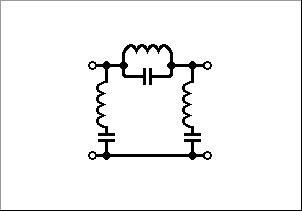A.   A high-pass filter
B.   A band-stop filter
C.   A band-pass filter
D.   A low-pass filter

And now for the answer to our May 23, 2018 TechNet Puzzle

(It is in “Invisotext” and will be visible if you highlight the area below!)

The best approach, unless you can recognize filter circuits is to consider how tuned LC circuits work. LC circuits with their capacitor and inductor in series will conduct through them the most at their resonant frequency. From the diagram above that would appear as a short circuit at the resonant frequency. When the inductor and capacitor are wired in parallel it conducts the least at its resonant frequency. The bottom line is that at this filter’s resonant frequency Its serial current is being impeded, while the signal is being shorted to ground. A high-pass filter would only block frequencies below the resonant frequency, a low-pass filter would only block frequencies above the resonant frequency. A band-pass filter would pass frequencies around the resonant frequency. Only a band-stop filter, or a notch filter, block frequencies near its resonant frequency.

So, the correct answer is “B” – a band-stop filter!

## The KCRC TechNet Puzzle for May 9, 2018

If you were going to construct a trap Yagi antenna, that would have three elements, and operate on three bands, how many taps would you need?

A.    6
B.    8
C.    9
D.   12

And now for the answer to our May 9, 2018 TechNet Puzzle
(It is in “Invisotext” and will be visible if you highlight the area below!)

Just think of it as a simple dipole x 3 (and don’t worry that one is a director and the other is a reflector right now.

A trap dipole for two bands would require a pair of trap dipoles for either side of the dipole. By using two taps on each side, you could tune the dipole for three bands. So that’s four traps for the single dipole. Now add the four traps for the reflector element and another four traps for the director element and you have 12 traps!

## The KCRC TechNet Puzzle for April 25, 2018

To receive SSB, the carrier insertion oscillator should be connected to which point?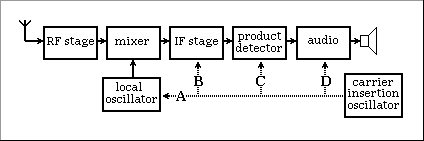A.   Point A
B.   Point B
C.   Point C
D.   Point D

.

And now for the answer to our April 25, 2018 TechNet Puzzle

(It is in “Invisotext” and will be visible if you highlight the area below!)

The way that Single Side Band is detected is by injecting a carrier tone onto a SSB signal and using a product detector to output an audio frequency signal. As an example, if you transmitted a 1,000 Hz pure tone on the upper sideband on 28.380 MHz, your output would appear to be a constant signal at 28.381 MHz. There is only one point in the diagram above where mixing an unmodulated carrier will result in an audio frequency signal that will not be filtered from its output, and that is point “C”.

## The KCRC TechNet Puzzle for April 11, 2018

A transmitter utilizes a balanced mixer to produce an SSB signal at 12MHz. To obtain an output at 144MHz the transmitter would

A    mix the signal with an oscillator output at 144MHz
B    use a frequency tripler followed by two frequency doublers.
C    mix the signal with another signal at 156MHz
D    feed the signal to a non-linear amplifier with a tuned collector load at 144MHz.

And now for the answer to our April 11, 2018 TechNet Puzzle

(It is in “Invisotext” and will be visible if you highlight the area below!)

For this question you have to recall how superheterodyne circuits work, both in receiver and transmitting stages. If you inject two different frequency signals through a nonlinear device, like a mixer, you get an output made up of the original two frequencies, as well as the sum and difference of those two original frequencies. If one of your signals has a modulating signal within it, all but the unmodulated carrier frequency mixed with the modulated signal will be free of the modulated signal.

If you tried answer “A” and mixed your 12 MHz modulated signal with a 144 MHz carrier signal, you would get a 122 MHz (144-12) modulated signal and a 156 MHz (144+12) signal, but your original 144 MHz carrier signal will continue to be unmodulated with no information contained within it! So, this is wrong!

If you tried answer “B” you might get to 144 eventually: 12 MHz x 3 (tripler) x 2 x 2 (doubler) does finally get you to 144 MHz BUT you will also be doubling and tripling your modulating signal as well – a pure 1,000 Hz tone modulating your original 12 MHz carrier will be demodulated as a 12,000 Hz signal – you would be received as though you lived in a very high pitched house filled with Helium! So, this one is wrong as well.

If you notice my discussion regarding answer “A” I mentioned that a carrier signal of 156 MHz when mixed with a modulated 12 MHz signal will result in a difference output of 144 MHz, so “C” seems quite correct!

Question “D” sounds interesting, but isn’t right either.

To reiterate, “C” is the correct answer!

.

## The KCRC TechNet Puzzle for March 28, 2018

Which factor below will have the greatest effect on the inductance of a coil with the same number of turns?

A    Inserting a glass rod inside the coil.
B    Increasing the current through the coil.
C    Increasing the diameter of the coil.
D    Reducing the diameter of the wire.

And now for the answer to our March 28, 2018 TechNet Puzzle
(It is in “Invisotext” and will be visible if you highlight the area below!)

For anyone interested, this is an approximate equation for an inductor’s inductance in Henries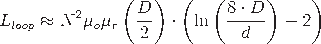Where N are the number of turns, D is the coil’s diameter, d is the wire’s diameter and the other odd-looking variables are relative permeability of the coil’s core.

Some choices are easier to reject than others. Choice “B” for any change of current is probably the easiest – current does NOT change an inductor’s inductance. Choice “A” is also relatively easy to reject. Strongly diamagnetic cores will decrease an inductor’s inductance, while highly paramagnetic, with a high permeability core, like a ferrite, will increase an inductor’s inductance, but a glass rod will have a very small effect upon an inductor’s inductance. Here’s where it might get a bit more difficult. Choice “B”, a coil’s diameter certainly influences an inductor’s inductance. To a degree choice “D” the diameter of the wire making the inductor also influences its inductance, but this relationship is far less than the length of the coil. The bottom line, is that given the above choices, the change that would have the greatest effect upon a given inductor’s inductance is choice “C” its coil diameter!

## The KCRC TechNet Puzzle for March 14, 2018

The maximum usable frequency is

A    usually within about 10% of the critical frequency
B    lower than the critical frequency but dependent on the actual path length
C    higher than the critical frequency and increases as the path length increases
D    higher than the critical frequency and decreases as the path length increases.

And now for the answer to our March 14, 2018 TechNet Puzzle

(It is in “Invisotext” and will be visible if you highlight the area below!)

Sorry, but you can’t use any Kung Fu logic on this question – you just must know a few definitions:

Maximum Usable Frequency (MUF) is “the highest radio frequency that can be used for transmission between two points via reflection/refraction from the ionosphere (skywave or “skip” propagation) at a specified time, independent of transmitter power. “

Critical Frequency is “the highest frequency reflected/refracted for a signal propagating directly upward.”

And how are they mathematically related?

Maximum Usable Frequency = Critical Frequency/cos θ

(Geezzz… not trigonometry?)

At θ of 0 degrees (straight up) cos θ is equal to 1 and MUF is the same as Critical Frequency, as you sweep θ across the horizon at bigger and bigger angles until you are getting near to the 90 degrees of the horizon, cos θ gets closer and closer to zero and the reciprocal of something getting closer and closer to zero gets very high! Obviously, this falls apart when your angle runs out of ionosphere, but that’s the principle.

So, as your path length increases, your θ increases and your MUF in relation to Critical Frequency increases. The answer that reflects this relationship is answer C “higher than the critical frequency and increases as the path length increases”, and “C” is the correct answer for this question!

## The KCRC TechNet Puzzle for February 28, 2018

The field strength from a UHF antenna is measured at a distance of 20m over clear ground. At twice that distance the measurement will be

A   much the same value
B  about half the first value
C  approximately quartered
D  impossible to predict.

And now for the answer to our February 28, 2018, TechNet Puzzle

(It is in “invisotext” and will be visible if you highlight the area below!)

Now, I introduced this as a “trick” question from the very beginning, so I hope that no one ran for the obvious, wrong answer…

In electromagnetic waves, which radio waves are an example of, power diminishes as an inverse square law. The spot of light striking a nearby wall from a flashlight extended twice the original distance will diminish its light to ¼ (1/22), and if extended three times its original distance, would diminish its light to 1/8 (1/23). Unfortunately, field strength isn’t the same as power. Field strength decreases by an inverse linear law. Twice the distance produces half the field strength.

RF power can be expressed as Watts per meter2, while field strength can be measured in volts per meter. It is very similar to how voltage and watts are seen to be related in a simple resistive circuit – double the resistance and the power in watts is decreased to one quarter, while the voltage is only decreased to one half.

Once you understand that little wrinkle you can see that the correct answer is B “about half the value”.

## The KCRC TechNet Puzzle for February 14, 2018

An antenna is quoted as having a Return Loss of 12dB at its center frequency. The transmission line to the shack has an attenuation of 2dB. The Return Loss measured in the shack will be

A    4dB
B    8dB
C  14dB
D  16dB

And now for the answer to our February 14, 2018 TechNet Puzzle

(It is in “Invisotext” and will be visible if you highlight the area below!)

You may want to look up what “Return Loss” really means – it is the ratio of power loss going from radio to antenna system and BACK AGAIN.

So, you have the 14 dB loss of the antenna, but what about the transmission line loss?
Well, it says 2 dB loss, so 14 + 2 = 14, right?

Ah, but you forgot about the “BACK AND FORTH” part – it’s 2 dB “BACK” and 2 dB “Forth”, for a total of 4 dB return loss for the transmission line.

So, it isn’t 12 + 2, it is 12 + 4 = 16

“D” 16 dB is the correct answer!

## The KCRC TechNet Puzzle for January 24, 2018

What does this interesting bit of electronics in the middle look like to you (please don’t just “Google this all at first – think about it for just a while)?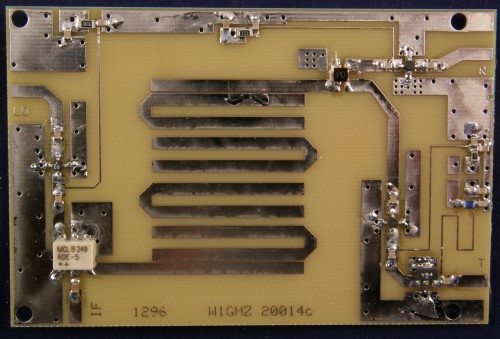Is this a…

A   Copper Snake
B   Hairpin Filter
C   Bias-T
D   Copper T

(and what the heck might it do????)

And now for the answer to our January 24, 2018 TechNet Puzzle

(It is in “Invisotext” and will be visible if you highlight the area below!)

This one might be difficult to “logic” into the answer alone unless your hobbies are Herpetology and Gynecology <grin>…

“A” Copper Snake, ain’t a euphemism, it’s just a variety of snake – and the illustration is a quite inorganic, nonliving printed circuit board, so this one is out!

“B” Hairpin Filter, is a real “electronics” thing, it is a close relative to a technique called “stripline”, where patterns designed for printed circuit boards can be used as actual components, rather than the wiring that usually connects everything together. Hairpin filters are primarily used in VHF/UHF and microwave. There are serpentine patterns of copper foil, designed into the printed circuit board to act as RF filters – The picture looks a LOT like that, so I think we might have “a keeper” here!

“C” Bias-T is an interesting little circuit that I am sure you have many examples of in a lot of your electronics. Basically, it is a DC/RF Diplexer – you can inject both currents in one port and by way of a DC blocking capacitor and an RF blocking inductor extract the two separate currents out the other end: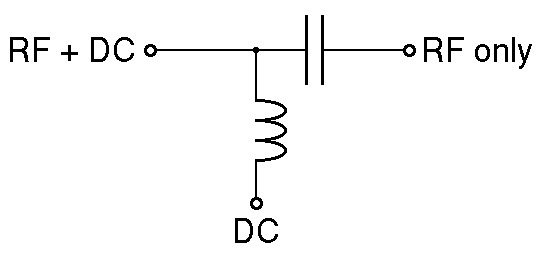Now, that’s all fascinating (at least to me), but that schematic looks nothing like the question’s photo, so it’s not a Bias-T circuit!

“D” Copper T, brings us to the amazing world of Gynecology and contraceptives, in particular, bundled together into the term “intrauterine device (IUD)”. Since this is not a GynNet, or even a BioNet, we’ll leave it as an exercise for the curious to learn further about this non-electronic technology.

So, we look in our back pocket for the possible answer that we haven’t thrown away and we get “B” Hairpin Filter!

## The KCRC TechNet Puzzle for January 10, 2018

Over-driving a power amplifier will…

A     give a high SWR
B     give minimum distortion on receive
C     generate excessive harmonics
D     maximize the power output

And now for the answer to our January 10, 2018, TechNet Puzzle

(It is in “invisotext” and will be visible if you highlight the area below!)

Let’s take your choices one at a time:

A) SWR (Voltage Standing Wave Ratio) is a consequence of an impedance mismatch in your antenna system. Although the calculated percentage of reflected power, that many SWR meters use to calculate your SWR is affected, to a degree by your final power output, overdriving your final power output should have no effect upon your antenna system’s SWR, so this one is wrong!

B) Overdriving your final power output stage should only affect your transmissions, not your reception (they are separate circuits inside your transceiver’s block diagram), so this one is wrong, too.

C) This one is a quite reasonable answer, so let’s hold on to this one!

D) Although overdriving your final power amplifier MAY lead to a great power output, it does not necessarily accomplish this (it depends upon the circuit and component choices, so this one very “iffy”

Power amplifiers are usually designed to squeeze as much power out of a design as possible – it’s just good economics, people like MORE power. The problem is not only that a circuit has a maximum gain/maximum output, you are also trying to use your circuit at a power level where it produces a LINEAR response gain. Past this region of LINEARITY, you get a very bad NON-LINEAR response that not only generates distortion on your intended frequency but generates so much distortion that it “leaks” through your amplifier’s filters, to blanket other frequencies with your splatter/noise. This used to be a BIG problem with some CB-ers – they would have their final amplifier input resistor’s resistance decreased, increasing the circuit’s gain, at the price of non-linearity, distortion and out of frequency emissions, but hey the wattmeter looked great!

As you have gleaned by now, the correct answer is/was “C”, excessive harmonic noise!